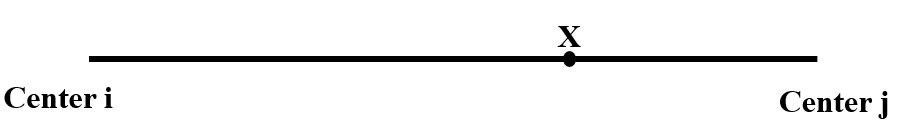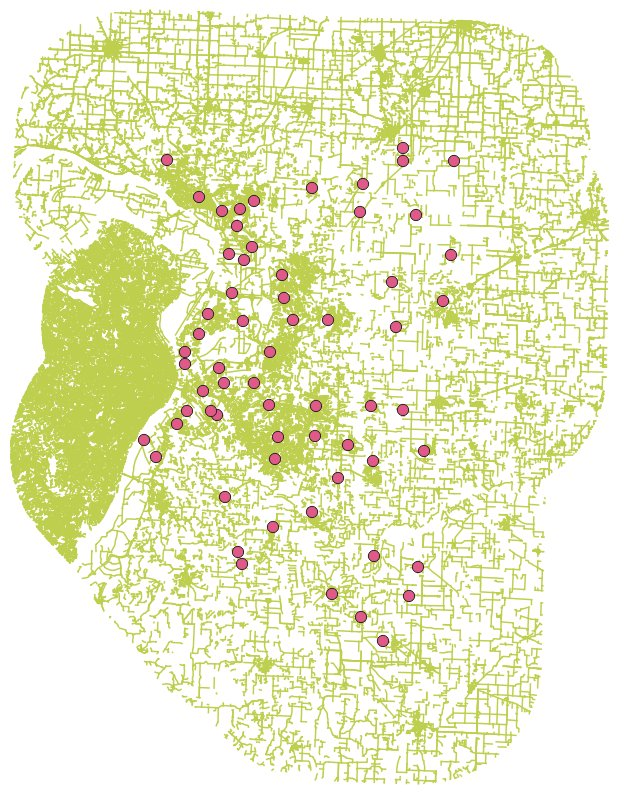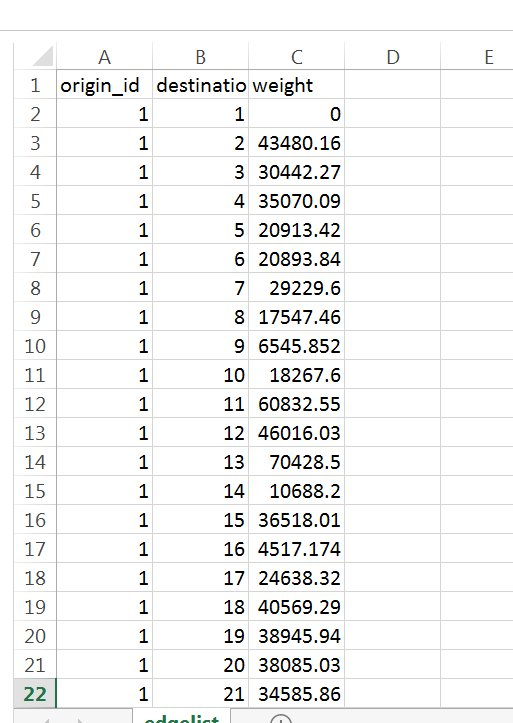### The standard Reilly’s law of retail gravitation

A classical gravity model can be expressed as

Tij=k Mi Mj/dij2

where Tij is the interaction (trade, commuting, etc.) between locations i and j; k a constant; Mi and Mj are masses (population, total income, etc.) of locations i and j respectively; and dij the distance between i and j.

According to the gravity model, a retail center’s attraction to its customers is Mi /di2 or Mj /dj2.

When the attractions from the two centers are equal, there is Mi /di2 = Mj /dj2Fig. 1 Market delineation between centers i and j

In Fig. 1, point X shows the location where attractions from the two centers, i and j, are equal. Thus, to the left of X, attraction from i is higher and customers shop at i. The opposite is true to the right of X. Clearly, X delineates market between centers i and j.

From Mi /di2 = Mj /dj2, it can be shown that d iX = dij/(1+ √Mj/Mi). Where diX is the distance from center i to the market boundary X. Thus the market boundary from center j to X is djX=dij-diX.

The purpose of Reilly’s law of retail gravilation is to find diX or djX while other conditions (dij, Mi, and Mj) are known.

### Reilly’s law of retail gravitation in two dimensional space

The standard Reilly’s model is one dimensional. A meaningful extension is to apply the principle to two dimensional space. As a result, several factors need to be considered.

First, while the one dimensional linear market is also the travel path, a two dimensional space requires a road network.

Second, customers travel along the road network to a given set of centers to purchase goods or services. Suppose they prefer to purchase at the nearest center. There is a need to calculate the pairwise market divides where the attractions from different centers are equal.

Third, there should be visualization of the market areas on the two dimensional space based on the principle of Reilly’s model.

The work-flow is indicated in Fig. 2. While the writing states what needs to be done at each step, the red symbol suggests the software to use for that step.Fig. 2 Work-flow for two dimensional Reilly’s model

#### 2. Calculate distance matrix over the centers

After the road network is obtained, there may be need for processing such as defining speed, directionality, or crossings. This could be a time-consuming process and will not be further discussed at this point. Assume the road network is ready, the distance matrix can be calculated using the QNEAT3 plugin of QGIS 3.4, which actually calculates an edge list. The edge list can be converted to distance matrix by R package igraph .

#### 3. Calculate market boundaries among pairwise centers

This step uses the R package SpatialPosition . Specifically, the reilly( ) function from the package is used with the following input: the center point shapefile with an attribte variable indicating the center masses (size measures such as population, total GDP, etc.), a outline shapefile for the study area, and distance matrix. The user also need to specify the distance decay function (power or exponential), the exponent of distance decay function, etc.

#### 4. Processing the results for visualization

Results from R package SpatialPosition needs to be further processed for clarity and visual appeal by using a few other R packages raster, rmapshaper, and rgeos . The final results include both raster and vector maps.

### Example 1: Reilly’s law of retail gravitation in two dimensional space

In this exmple, I used a point shapefile called places_2county (Fig. 3), which contains places and designated places defined by the U.S. Bureau of Census in the Madison and St. Clair two county region of Illinois; a line shapefile roads_2county (Fig. 3), contains roads within the bi-county area with about a 19 km (11 miles) buffer of roads; the third file is a polygon shapefile which is the outline of the Madison and St. Clair two county region. This last file is used for reilly( ) function.Fig. 3 Centers and road network                     Fig. 4 A portion of the edge list, distance in meters